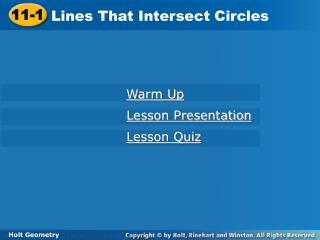DownloadDownload Presentation11-1

# 11-1

Download Presentation## 11-1

- - - - - - - - - - - - - - - - - - - - - - - - - - - E N D - - - - - - - - - - - - - - - - - - - - - - - - - - -
##### Presentation Transcript

1. 11-1 Lines That Intersect Circles Warm Up Lesson Presentation Lesson Quiz Holt Geometry

2. Objectives Identify tangents, secants, and chords. Use properties of tangents to solve problems.

3. Vocabulary interior of a circle point of tangency exterior of a circle tangent circles chord common tangent secant tangent of a circle

4. This photograph was taken 216 miles above Earth. From this altitude, it is easy to see the curvature of the horizon. Facts about circles can help us understand details about Earth. Recall that a circle is the set of all points in a plane that are equidistant from a given point, called the center of the circle. A circle with center C is called circle C, or C.

5. JM and KM JM LK, LJ, and LM KM Example 1: Identifying Lines and Segments That Intersect Circles Identify each line or segment that intersects L. chords: secant: tangent: diameter: radii: m

6. QR and ST ST PQ, PT, and PS ST UV Check It Out! Example 1 Identify each line or segment that intersects P. chords: secant: tangent: diameter: radii:

7. A common tangent is a line that is tangent to two circles.

8. A common tangent is a line that is tangent to two circles.

9. 1 Understand the Problem Example 3: Problem Solving Application Early in its flight, the Apollo 11 spacecraft orbited Earth at an altitude of 120 miles. What was the distance from the spacecraft to Earth’s horizon rounded to the nearest mile? The answer will be the length of an imaginary segment from the spacecraft to Earth’s horizon.

10. Make a Plan Draw a sketch. Let C be the center of Earth, E be the spacecraft, and H be a point on the horizon. You need to find the length of EH, which is tangent to C at H. By Theorem 11-1-1, EH CH. So ∆CHE is a right triangle. 2

11. 3 Solve EC = CD + ED Seg. Add. Post. Substitute 4000 for CD and 120 for ED. = 4000 + 120 = 4120 mi Pyth. Thm. EC2 = EH² + CH2 Substitute the given values. 41202 = EH2+ 40002 Subtract 40002 from both sides. 974,400 = EH2 Take the square root of both sides. 987 mi  EH

12. 4 Look Back The problem asks for the distance to the nearest mile. Check if your answer is reasonable by using the Pythagorean Theorem. Is 9872 + 40002 41202? Yes, 16,974,169  16,974,400.

13. 1 Understand the Problem Check It Out! Example 3 Kilimanjaro, the tallest mountain in Africa, is 19,340 ft tall. What is the distance from the summit of Kilimanjaro to the horizon to the nearest mile? The answer will be the length of an imaginary segment from the summit of Kilimanjaro to the Earth’s horizon.

14. Make a Plan Draw a sketch. Let C be the center of Earth, E be the summit of Kilimanjaro, and H be a point on the horizon. You need to find the length of EH, which is tangent to C at H. By Theorem 11-1-1, EH CH. So ∆CHE is a right triangle. 2

15. 3 Solve Given ED = 19,340 Change ft to mi. EC = CD + ED Seg. Add. Post. = 4000 + 3.66 = 4003.66mi Substitute 4000 for CD and 3.66 for ED. EC2 = EH2 + CH2 Pyth. Thm. Substitute the given values. 4003.662 = EH2+ 40002 Subtract 40002 from both sides. 29,293 = EH2 Take the square root of both sides. 171  EH

16. 4 Look Back The problem asks for the distance from the summit of Kilimanjaro to the horizon to the nearest mile. Check if your answer is reasonable by using the Pythagorean Theorem. Is 1712 + 40002 40042? Yes, 16,029,241  16,032,016.

17. HK and HG are tangent to F. Find HG. Example 4: Using Properties of Tangents 2 segments tangent to from same ext. point segments . HK = HG 5a – 32 = 4 + 2a Substitute 5a – 32 for HK and 4 + 2a for HG. 3a –32 = 4 Subtract 2a from both sides. 3a = 36 Add 32 to both sides. a = 12 Divide both sides by 3. HG = 4 + 2(12) Substitute 12 for a. = 28 Simplify.

18. RS and RT are tangent to Q. Find RS. Substitute for RS and x – 6.3 for RT. x 4 Check It Out! Example 4a 2 segments tangent to from same ext. point segments . RS = RT x = 4x – 25.2 Multiply both sides by 4. –3x = –25.2 Subtract 4x from both sides. x = 8.4 Divide both sides by –3. Substitute 8.4 for x. = 2.1 Simplify.

19. RS and RT are tangent to Q. Find RS. Check It Out! Example 4b 2 segments tangent to from same ext. point segments . RS = RT Substitute n + 3 for RS and 2n – 1 for RT. n + 3 = 2n –1 4 = n Simplify. RS = 4 + 3 Substitute 4 for n. = 7 Simplify.

20. chords VT and WR secant: VT tangent: s diam.: WR radii: QW and QR Lesson Quiz: Part I 1. Identify each line or segment that intersects Q.

21. 4. FE and FG are tangent to F. Find FG. Lesson Quiz: Part III 3. Mount Mitchell peaks at 6,684 feet. What is the distance from this peak to the horizon, rounded to the nearest mile?  101 mi 90